# Parametric Excitation and Amplification of Electromagnetic

The following article is from The Great Soviet Encyclopedia (1979). It might be outdated or ideologically biased.

## Parametric Excitation and Amplification of Electromagnetic Oscillations

a method of exciting and amplifying electromagnetic oscillations. In this method, power amplification results from the expenditure of energy on the periodic variation of the magnitude of a reactance parameter (the inductance L or the capacitance C) in an oscillatory system. The possibility of parametric excitation and amplification of electromagnetic oscillations was first pointed out by L. I. Mandel’shtam and N. D. Papaleksi. Practical application of the parametric method, however, did not begin until the 1950’s, when parametric semiconductor diodes with a controllable capacitance were produced and low-noise parametric amplifiers were developed for microwave frequencies.

Our discussion of the principle of parametric amplification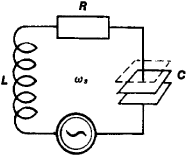Figure 1. Circuit with a periodically varying capacitance C; the value of the capacitance is equal to C0 when the capacitor plates are close together (solid lines) and to C1, when the plates are moved apart (broken line)

and generation will begin with a very simple example—an oscillatory circuit consisting of a fixed resistance R, a fixed inductance L, and a capacitance C that varies periodically with time (Figure 1). At resonance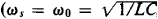where ws is the frequency of the signal to be amplified and w0 is the natural frequency of the circuit), the charge q on the plates of the capacitor varies according to the law

(1) q = q0 sin wst = CQℰ0 sin wst

Here, ℰo is the amplitude of the signal and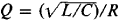is the quality factor of the circuit. The electrostatic energy W stored in the capacitor is equal to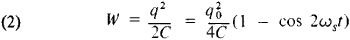It is evident from (2) that W varies at a frequency equal to twice the signal frequency. If the capacitance of C is changed abruptly by AC at the moment when q = q0 (for example, by moving the capacitor plates apart), the charge q will lack time to change but the energy W will be changed by the amount (if ∆C/C ≪ 1)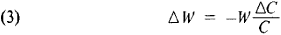Hence, for a C that varies periodically, the resulting energy increase in the circuit is a maximum if the capacitance is reduced when q is at a maximum and is restored to its initial value when q = 0. It follows that if C is varied at a frequency wp = 2ws in a certain phase (Figure 2), the device varying C will pump energy into the circuit twice in each oscillation period. If, on the other hand, C is increased when q is at a minimum, the circuit oscillations will be attenuated. The condition for effective pumping is given in a more general form by the equation wp = 2ws/n, where n = 1, 2, 3, …. When n = 1, C changes every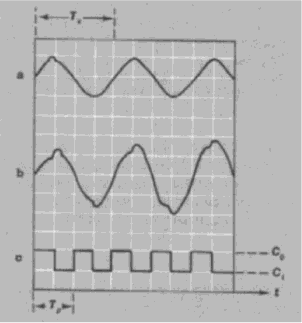Figure 2. Relationship between the variation of the voltage across a capacitance and the variation of the value of the capacitance: (a) voltage (of a signal undergoing amplification) across the capacitor when the value of the capacitance is fixed, (b) increase in signal voltage across the capacitor during parametric amplification, (c) capacitance variation during parametric amplification; (Ts) period of oscillation of the signal undergoing amplification, (Tp) period of oscillation of the pump signal

quarter of a signal period, that is, Ts/4. For larger values of n, C changes at time intervals equal to nTs/2.

A simple single-circuit parametric amplifier usually consists of an oscillatory system in which a harmonic voltage from a pump oscillator acts on a parametric semiconductor diode so as to vary the capacitance C; the diode’s capacitance is a function of the voltage applied to it. A parametric amplifier for microwave frequencies is made in the form of a four-way waveguide junction (Figure 3); the signal being amplified propagates along one of the waveguides and the pump signal along the other.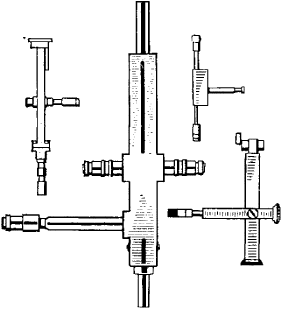Figure 3. Single-circuit parametric amplifiers

A parametric diode is located at the intersection of the waveguides. The power amplification factor is approximately equal to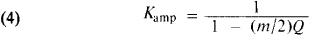where m = (Cmax - Cmin)/(Cmax + Cmin) is known as the depth of the capacitance variation. As (m/2)Q → 1, the amplification factor increases without limit; when (m/2)Q ≥ 1, the system turns into a parametric oscillator. A basic disadvantage of the single-circuit parametric amplifier is that Kamp depends on the relationship between the phases of the amplified signal and the pump signal.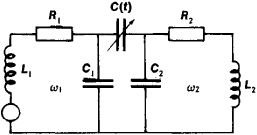Figure 4. Schematic of a two-circuit parametric amplifier

Parametric amplifiers with two or more circuits (Figure 4) do not have this disadvantage. In a two-circuit parametric amplifier the frequency and phase of the oscillations in the second, or idler, circuit are automatically adjusted so as to satisfy the conditions for the efficient pumping of energy. If the idler circuit is tuned to a frequency w2 = wpws, the pump energy is spent on amplifying the oscillations in both circuits; for this case,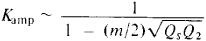When (m/2)√QSQ2 → 1, the amplifier turns into an oscillator. Such an amplifier is called regenerative. If the amplified signal is removed from the second circuit of a regenerative amplifier, the amplifier is also a frequency converter. When w2 = wp + ws, all the pump energy and the energy stored in the signal circuit become the energy of oscillations at the sum frequency (wp + ws. Such a parametric amplifier is called a nonregenerative amplifier-converter. It is stable for any m and has a broad pass-band but only a small Kamp.

In addition to the periodic variation of capacitance by means of parametric diodes, other types of parametric action are used. An inductance L can be varied periodically by using changes in the equivalent inductance of ferrites and superconductors. A periodic variation of the capacitance C can be obtained by making use of the dependence of the dielectric constant of dielectrics on the electric field, by using metal-oxide-semiconductor structures (surface varactors), or by other methods. The nonlinear properties of a density-modulated electron beam are made use of in electron beam-type parametric amplifiers.

In addition to resonator-type parametric amplifiers, there are traveling-wave parametric amplifiers, in which the electromagnetic signal wave propagates along a wave guide and interacts sequentially with each of the parametric diodes (or other nonlinear elements) positioned in the channel. The capacitance of the diodes is varied by means of pump energy supplied to the resonators. With the proper choice of frequencies, wavelengths, and directions of propagation for the pump and signal waves, the signal amplification increases exponentially as it propagates along the chain of diodes (Figure 5). A passband of up to 25 percent of the carrier frequency can be achieved in traveling-wave amplifiers—in contrast to a few percent for resonator-type amplifiers.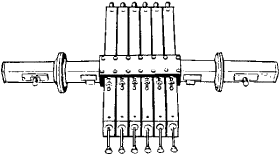Figure 5. Traveling-wave parametric amplifier

### REFERENCES

Mandel’shtam, L. I. Poln. sobr. trudov, vol. 2. Moscow-Leningrad, 1947.
Etkin, V. S. and E. M. Gershenzon. Parametricheskie sistemy SVCh na poluprovodnikovykh diodakh. Moscow, 1964.
Regenerativnye poluprovodnikovye parametricheskie usiliteli (nekotorye voprosy teorii i rascheta). Moscow, 1965.
Kaplan, A. E., Iu. A. Kravtsov, and V. A. Rylov. Parametricheskie generatory i deliteli chastoty. Moscow, 1966.
Lopukhin, V. M., and A. S. Roshal’. Elektronnoluchevye parametricheskie usiliteli. Moscow, 1968. V. I. ZUBKOV